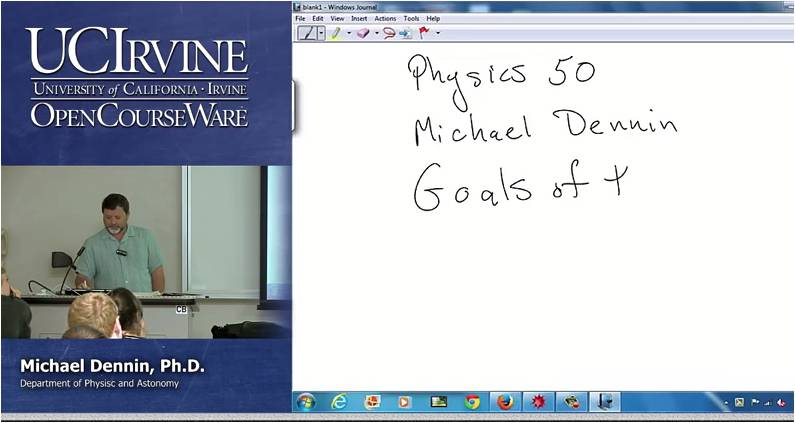# InfoCoBuild

## Physics 50 - Math Methods

Physics 50: Math Methods (Spring 2014, UC Irvine). Instructor: Professor Michael Dennin. Mathematica and its applications to linear algebra, differential equations, and complex functions. Fourier series and Fourier transforms. Other topics in integral transforms. Topics covered include: series representations for functions, complex numbers, matrices, vectors, tensors, abstract vector spaces, Fourier series and Fourier transformations, differential equations, derivatives and integrals, vector calculus, and partial differential equations.Lecture 01 - Introduction Lecture 02 - Series Representations for Functions, Complex Numbers Lecture 03 - Matrices Lecture 04 - Vectors Lecture 05 - Tensors Lecture 06 - Matrices II: Change of Basis, Diagonalization, Hermitian Matrix Lecture 07 - Abstract Vector Spaces Lecture 08 - 2-Dimensional Vector Spaces Lecture 09 - Fourier Series/ Fourier Transformations Lecture 10 - Fourier Series/ Fourier Transformations (cont.) Lecture 11 - Differential Equations (DEQs) Lecture 12 - Differential Equations (DEQs) (cont.) Lecture 13 - Derivatives and Integrals Lecture 14 - Derivatives and Integrals (cont.) Lecture 15 - Line Integrals Lecture 16 - Vector Calculus Lecture 17 - Vector Calculus (cont.) Lecture 18 - Partial Differential Equations Lecture 19 - Partial Differential Equations

 Related Links Physics 50: Math Methods Instructor: Professor Michael Dennin. Mathematica and its applications to linear algebra, differential equations, and complex functions. Fourier series and Fourier transforms. Other topics in integral.